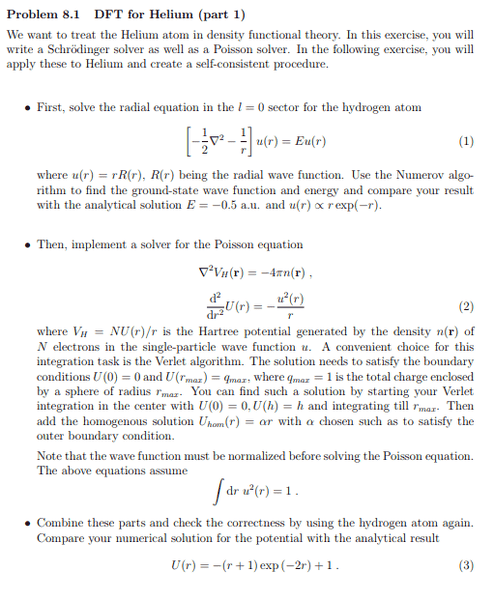# DFT for Helium using Mathematica

• Astr
In summary, the problem arises when vhartree is not zero. My program works perfect when vhartree={0,0,..,0}.f

#### Astr

I'm trying to solve this problem with Mathematica.Im not a Mathematica expert, but my program works perfect when vhartree={0,0,..,0}.

This is the program I wrote:
Code:
Clear[u, poisson, vhartree]
h = 10^(-2);(*step integration*)
rmax = 20;
rmin = 10^(-30);
Z = 2; (*atomic number*)
points = rmax/h;
vhartree = Table[0, {i, 1, points + 1}];
(*numerov integration*)
g[energy_, j_] :=
Module[{a},
If [j < rmax/h,
a = N[2 (energy + Z/(rmax - j h)) - vhartree[[points - j]]],
a = N[2 (energy + 1/rmin)] + vhartree[]];
Return[a];
];
numerov[energy_] := Module[{j},
u = {N[rmax*Exp[-rmax]]};
PrependTo[u, N[(rmax - h)*Exp[-(rmax - h)]]];
alpha = N[h^2/12];
x = u[];
y = u[];
z = (2 (1 - 5 alpha g[energy, 1]) y + (1 +
alpha g[energy, 0]) x)/(1 + alpha g[energy, 2]);
PrependTo[u, z];
For[ j = 2, j < points, j++,
x = y;
y = z;
z = (2 (1 - 5 alpha g[energy, j]) y - (1 +
alpha g[energy, j - 1]) x)/(1 + alpha g[energy, j + 1]);
PrependTo[u, z];
];
Return
];

searchenergy := Module[{k, n, a, b, M, j},
(*searching range where numerov[] change its sign*)
k = 1;
a = -10 ;(*min. energy*)
While[numerov[a][]*numerov[a + 0.1][] > 0,
a = a + 0.1;
k++;
];

b = a + 0.1; (*max energy*)
(*bisection*)
M = 50;(*max number of iterations*)
wave = {};
n = 0;
epsilon = 10^(-8);
While[(n < M) && (Abs[a - b] > epsilon),
energy = N[(a + b)/2];
If[(numerov[a][])*(numerov[energy][]) > 0, a = energy,
b = energy];
n++;
];
wave = numerov[energy];
(*normalization, Simpson's rule*)
hsimpson = rmax/(Length[wave]);
integrate = (hsimpson/3) ((wave[])^2 +
2 Sum[wave[[2 j]]^2, {j, 1, Length[wave]/2 - 1}] +
4 Sum[wave[[2 j - 1]]^2, {j, 1, Length[wave]/2}]);
wave = wave/Sqrt[integrate];
Return[wave];
]
Poisson := Module[{i, beta, t, v, w},
(*Verlet algorithm*)
t = 0;
v = h;
poisson = {t, v};
For[i = 1, i <= puntos - 1, i++,
w = 2 v - t - (h) wave[[I]]^2/(i);
AppendTo[poisson, w];
t = v;
v = w;
];
beta = (1 - poisson[[Length[poisson]]])/rmax;
For[i = 1, i <= Length[poisson], i++,
poisson[[I]] = poisson[[I]] + beta (i - 1) h;
];
Return[poisson];
]
Clear[k]
lista = {energy};
For[k = 0, k < 5, k++,
vhartree[] = poisson[]/rmin;
For[m = 2, m <= Length[poisson], m++,
vhartree[[m]] = 2 poisson[[m]]/((m + 1) h);
];
(*print the number of cycles before self consistency*)
Print[k];
searchenergy;
AppendTo[lista, energy];
Poisson;
]
integrate2 = (hsimpson/3) *(vhartree[] (wave[])^2 +
vhartree[[Length[vhartree]]] (wave[[Length[vhartree]]])^2 +
2 Sum[vhartree[[2 j]] (wave[[2 j]])^2, {j, 1,
Length[vhartree]/2 - 1}] +
4 Sum[vhartree[[2 j - 1]] (wave[[2 j - 1]])^2, {j, 1,
Length[vhartree]/2}]);
2energy-integrate2[/I][/I][/I]

The problem arises when vhartree is not zero. In books: 2energy-integrate2= -2.861, I get: -3.89561. I would appreciate any suggestion, thanks in avance for your help. In this page, you can find the code in Python for the whole problem (including the exchange potential):

<https://www.leetspeak.org/teaching/cqp_fs14/09-dft.html>

#### Attachments

Last edited by a moderator:
@Astr, please repost your code. The mentors have tried to format it so that it is readable, but there are still problems, mostly due to browsers mistakenly interpreting array or list indexes of 'i' as the start tag for BBCode italics.

In your opening post (now modified by mentor actions), the text changed from regular text to Italics in your module for the Verlet algorithm. This was caused by writing wave[i]. To prevent this, add a space before the i index like this: wave[ i], or use another index, like j or k.

If you are getting results that you don't expect, that's where debuggers are extremely useful. I am not at all familiar with Mathematica, so don't know what debugging capabilities it has. Since you're not getting the expected result from "2energy-integrate2" I would look at each of these expressions to see what values they have. BTW, I don't believe "2energy" is a valid expression. Mathematica, unlike virtually all other programming languages, allows you to omit an explicit multiplication operator, but I believe you have to at least include a space.

Hello @Mark44, thank you for your reply. I translate my program from spanish and I missed a ""puntos"" where should be changed for "points" , I wanted to edited but I don't know how.

By the way, In mathematica 2energy=2*energy is a valid operation, no need for whitespaces, though integrate2 is a valid variable name.